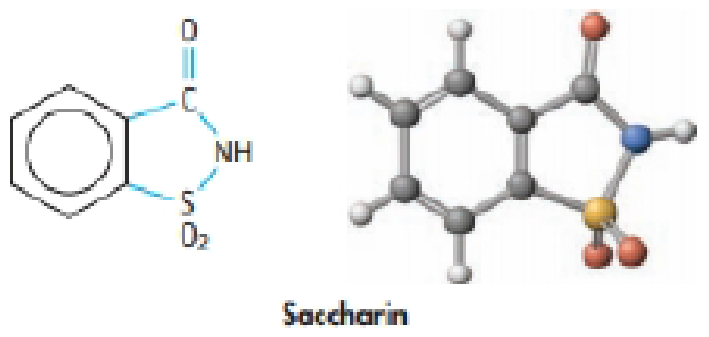Chapter 16, Problem 99GQ

Chapter
Section
Textbook Problem

Saccharin (HC7H4NO3S) is a weak acid with pKa = 2.32 at 25 °C. It is used in the form of sodium saccharide, NaC7H4NO3S. What is the pH of a 0.01M solution of sodium saccharide at 25 °C?Interpretation Introduction

Interpretation:

The pH of a 0.10M solution of sodium saccharide at 25oC has to be determined.

Concept introduction:

Equilibrium constants:

The equilibrium constant is used to quantitative measurement of the strength of the acid and bases in the water.

Ka is an acid constant for equilibrium reactions.

HA + H2OH3O++ A-Ka[H3O+][A-][HA]

Kb is base constant for equilibrium reaction.

BOH + H2OB++ OH-Ka[B+][OH-][BOH]

Ion Product constant for water

Kw= [H3O+][OH-]       =1.00×10-14 pH = -log[H3O+]pOH= -log[OH-]

Relation between pH and pOH

pH + pOH =14

Explanation

The given pKa of saccharine HC7H4NO3S is 2.32.

Pka = -logKa2.32 = -logKaa= 10-2.32Ka = 4.8×10-3

Let’s calculate the Kb of saccharine.

Kw= Ka×Kb

Kb of C7H4NO3S- = KwC7H4NO3S-                              = 1.0×10-144.8×10-3                              = 2.1×10-12Kb of C7H4NO3S- is 2.1×10-12

The equilibrium chemical reaction is as follows.

C7H4NO3S-(aq) + H2O(l)HC7H4NO3S(aq) + OH-(aq)

The equilibrium expression:

Kb[HC7H4NO3S][OH-][C7H4NO3S-]

The initial concentration =0.10

Enter the ICE table the concentrations before equilibrium is established, the change that occurs as the reaction proceeds to equilibrium and the concentrations when equilibrium has been achieved

C7H4NO3S-(aq) + H2O(l)HC7H4NO3S(aq) + OH-(aq)I         0.10M                  --                    --                      --C           -x                      --                  + x                   + xE        (0

Still sussing out bartleby?

Check out a sample textbook solution.

See a sample solution

The Solution to Your Study Problems

Bartleby provides explanations to thousands of textbook problems written by our experts, many with advanced degrees!

Get Started

What is the structure and function of a mitochondrion?

Biology: The Dynamic Science (MindTap Course List)

How can solar flares affect Earth?

Horizons: Exploring the Universe (MindTap Course List)

List the differences between mitosis and meiosis in the following chart:

Human Heredity: Principles and Issues (MindTap Course List)

List three common substances that will sublime.

Chemistry for Today: General, Organic, and Biochemistry